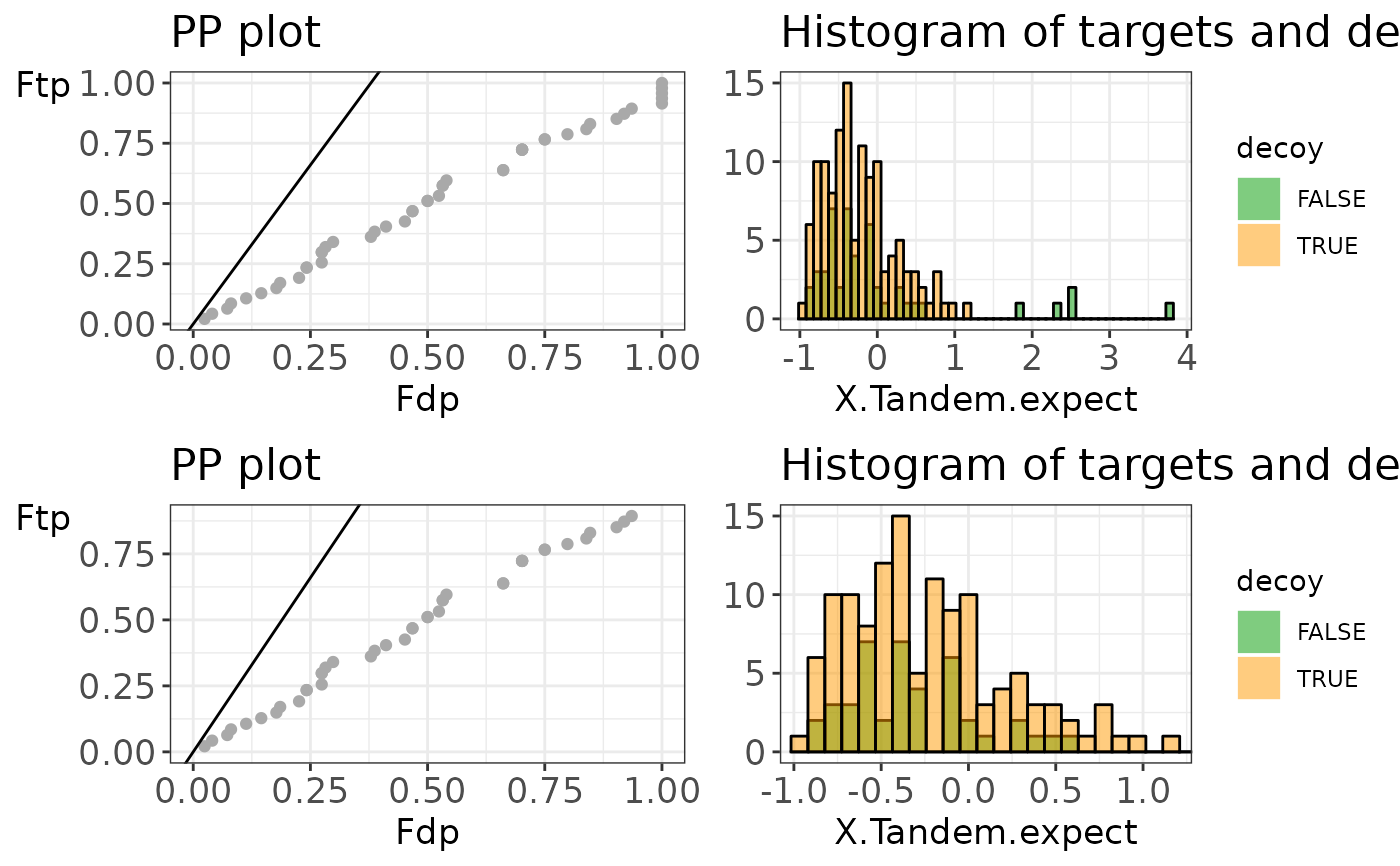Create diagnostic plots to evaluate the TDA assumptions. A histogram and PP plot allow to check both necessary assumptions.

evalTargetDecoys(
object,
decoy = NULL,
score = NULL,
log10 = TRUE,
nBins = 50,
maxPoints = 1000
)

evalTargetDecoysPPPlot(
object,
decoy = NULL,
score = NULL,
log10 = TRUE,
zoom = FALSE,
maxPoints = 1000
)

evalTargetDecoysHist(
object,
decoy = NULL,
score = NULL,
log10 = TRUE,
nBins = 50,
zoom = FALSE
)

## Arguments

object

A data.frame, mzID or mzRident object.

decoy

character, name of the variable that indicates if the peptide matches to a target or to a decoy. When this value is missing, a Shiny gadget is launched to select it interactively.

score

numeric, indicating the score of the peptide match, obtained by the search engine. When this value is missing, a Shiny gadget is launched to select it interactively.

log10

logical to indicate if the score should be -log10-transformed. Default: TRUE. When this value is missing, a Shiny gadget is launched to select it interactively.

nBins

numeric indicating the number of bins in the histogram. When this value is missing, a Shiny gadget is launched to select it interactively.

maxPoints

numeric indicating the maximum number of dots shown in the PP plot. If maxPoints is larger than the number of target scores, a dot in the PP plot corresponds with each target score in the object. The default is 1000 points.

zoom

Logical value indicating whether a zoomed version of the plot should be returned. Default: FALSE.

## Value

evalTargetDecoys returns an overview of the following four plots:

1. A PP plot showing the empirical cumulative distribution of the target distribution in function of that of the decoy distribution

2. A histogram showing the score distributions of the decoys and non-decoys

3. A zoomed PP plot

4. A zoomed histogram

evalTargetDecoysPPPlot generates the PP plot only (1.) or the zoomed version (3.) if zoom = TRUE.

evalTargetDecoysHist generates the histogram only (2.) or the zoomed version (4.) if zoom = TRUE.

Sometimes the variable names are not known up front. If this is the case, the evalTargetDecoys*() functions can be called with only an input object. This launches a Shiny gadget that allows selecting the variables interactively. A histogram and PP-plot of the selected variables are created on the fly for previewing, together with a snapshot of the selected data.

## Author

Elke Debrie, Lieven Clement, Milan Malfait

## Examples

library(mzID)

## Use one of the example files in the mzID package
exampleFile <- system.file("extdata", "55merge_tandem.mzid", package = "mzID")
mzIDexample <- mzID(exampleFile)

# Plot the overview of the four plots
evalTargetDecoys(mzIDexample,
decoy = "isdecoy", score = "x\\!tandem:expect", log10 = TRUE
)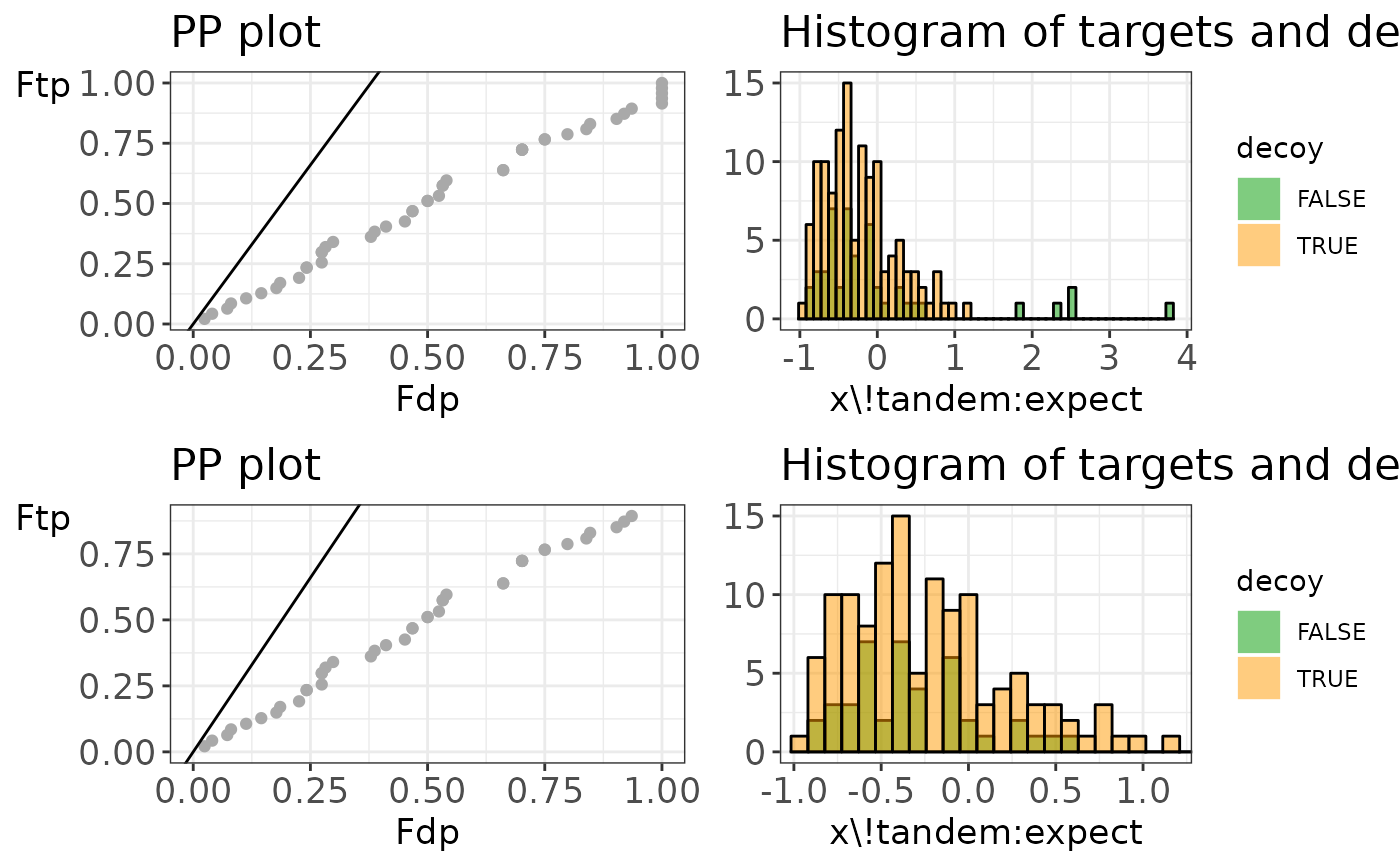# Plot the PP plot only
evalTargetDecoysPPPlot(mzIDexample,
decoy = "isdecoy", score = "x\\!tandem:expect", log10 = TRUE
)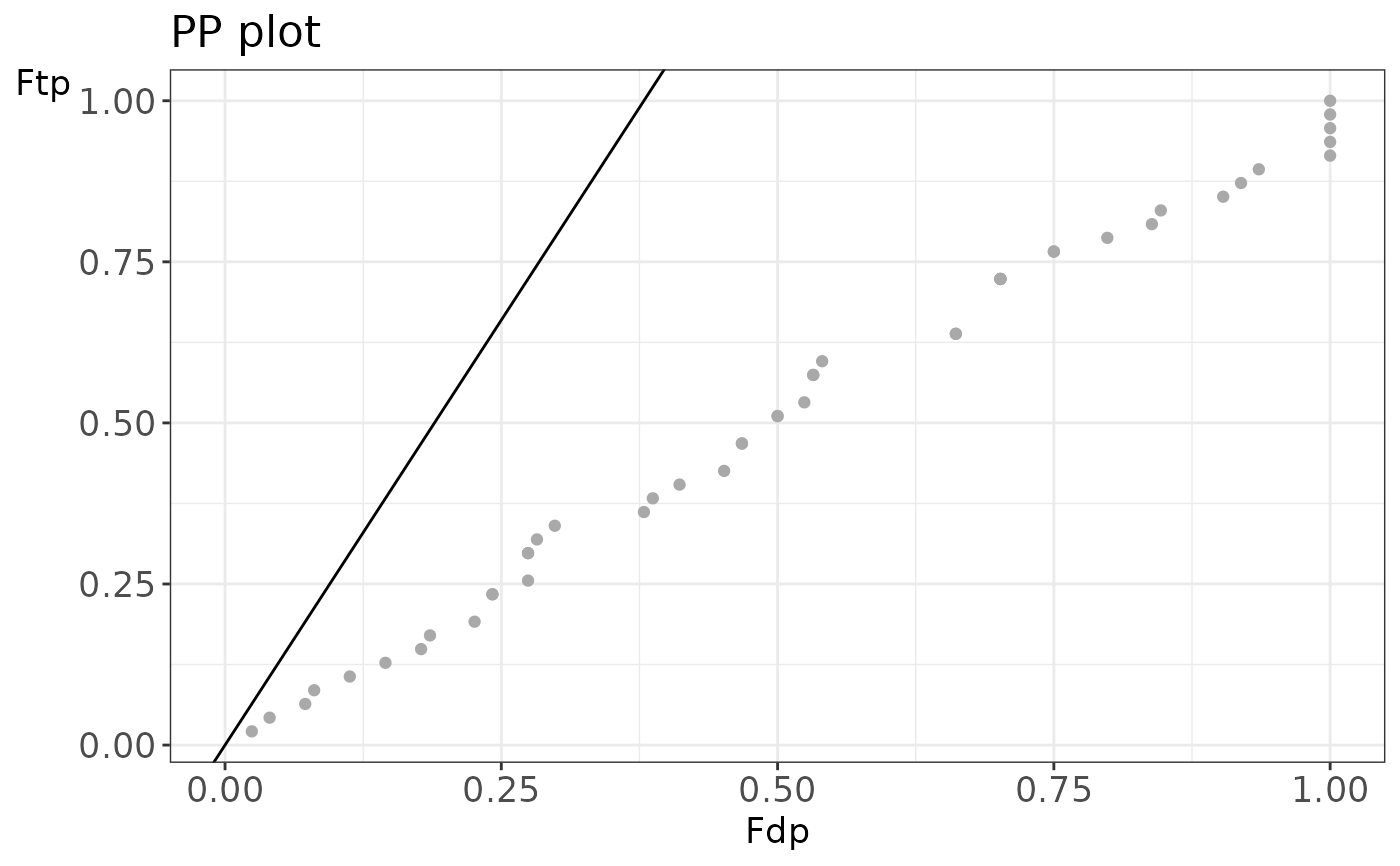# Plot the zoomed PP plot only
evalTargetDecoysPPPlot(mzIDexample,
decoy = "isdecoy", score = "x\\!tandem:expect", log10 = TRUE,
zoom = TRUE
)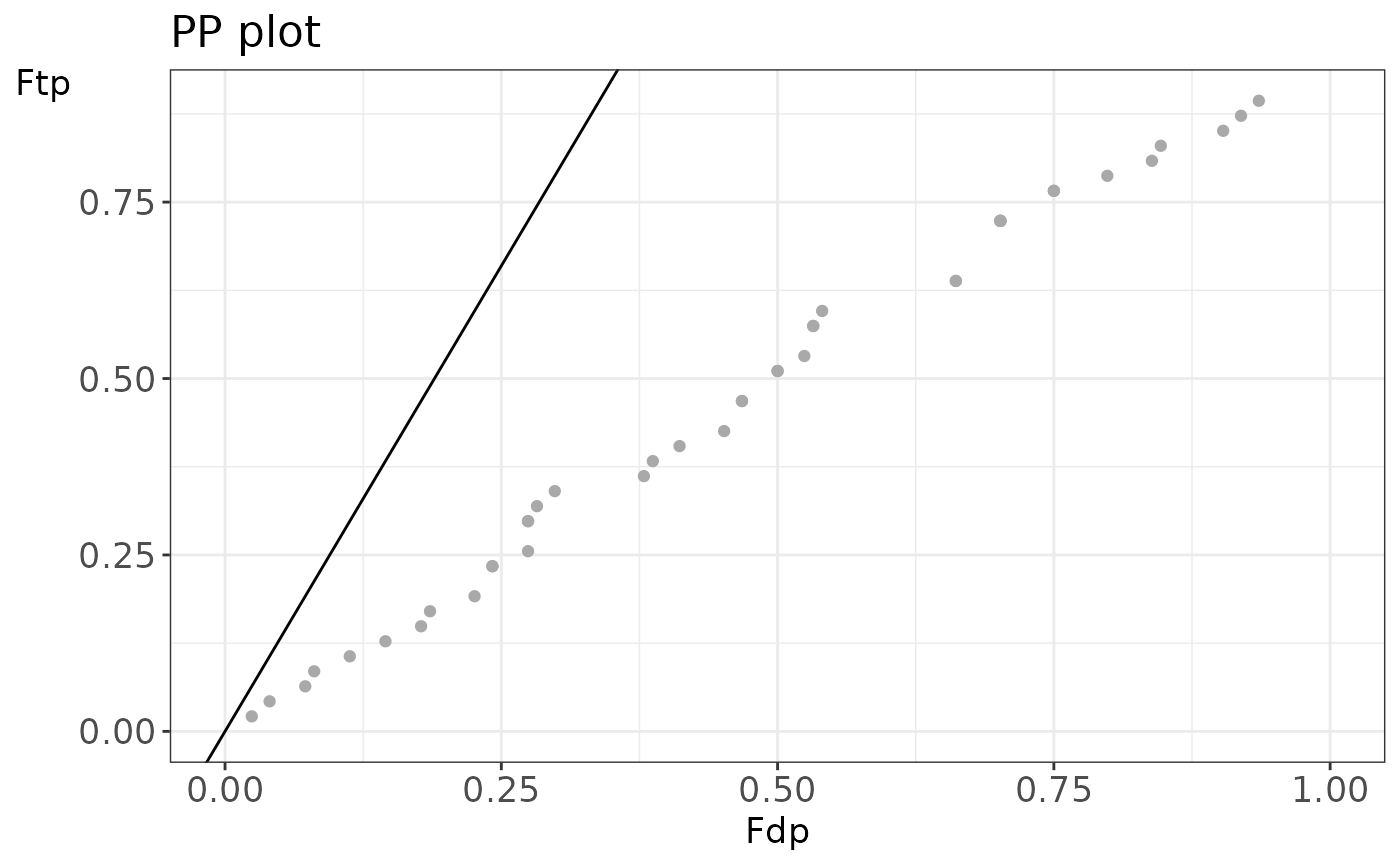# Plot the histogram only
evalTargetDecoysHist(mzIDexample,
decoy = "isdecoy", score = "x\\!tandem:expect", log10 = TRUE
)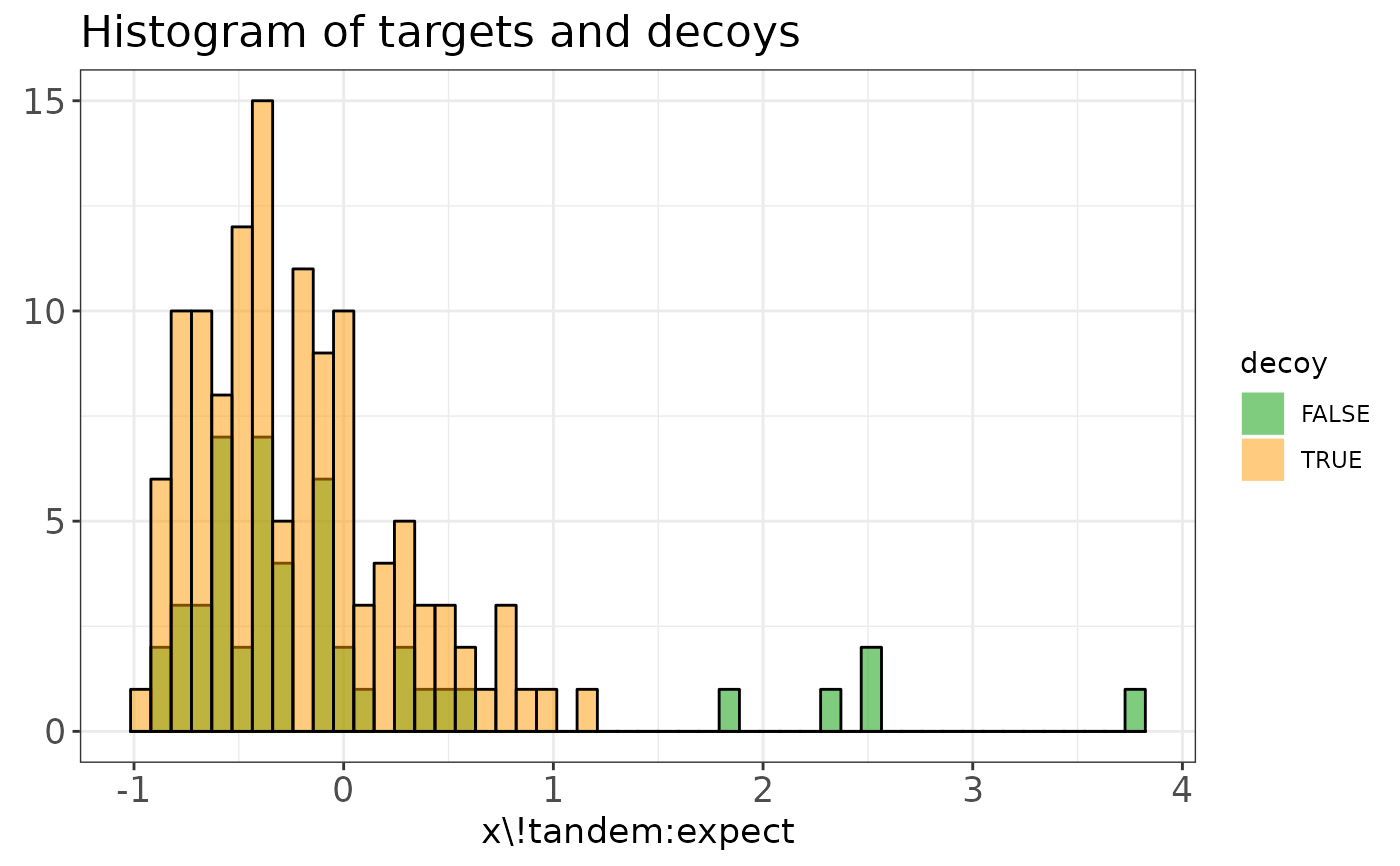# Plot the zoomed histogram only
evalTargetDecoysHist(mzIDexample,
decoy = "isdecoy", score = "x\\!tandem:expect", log10 = TRUE,
zoom = TRUE
)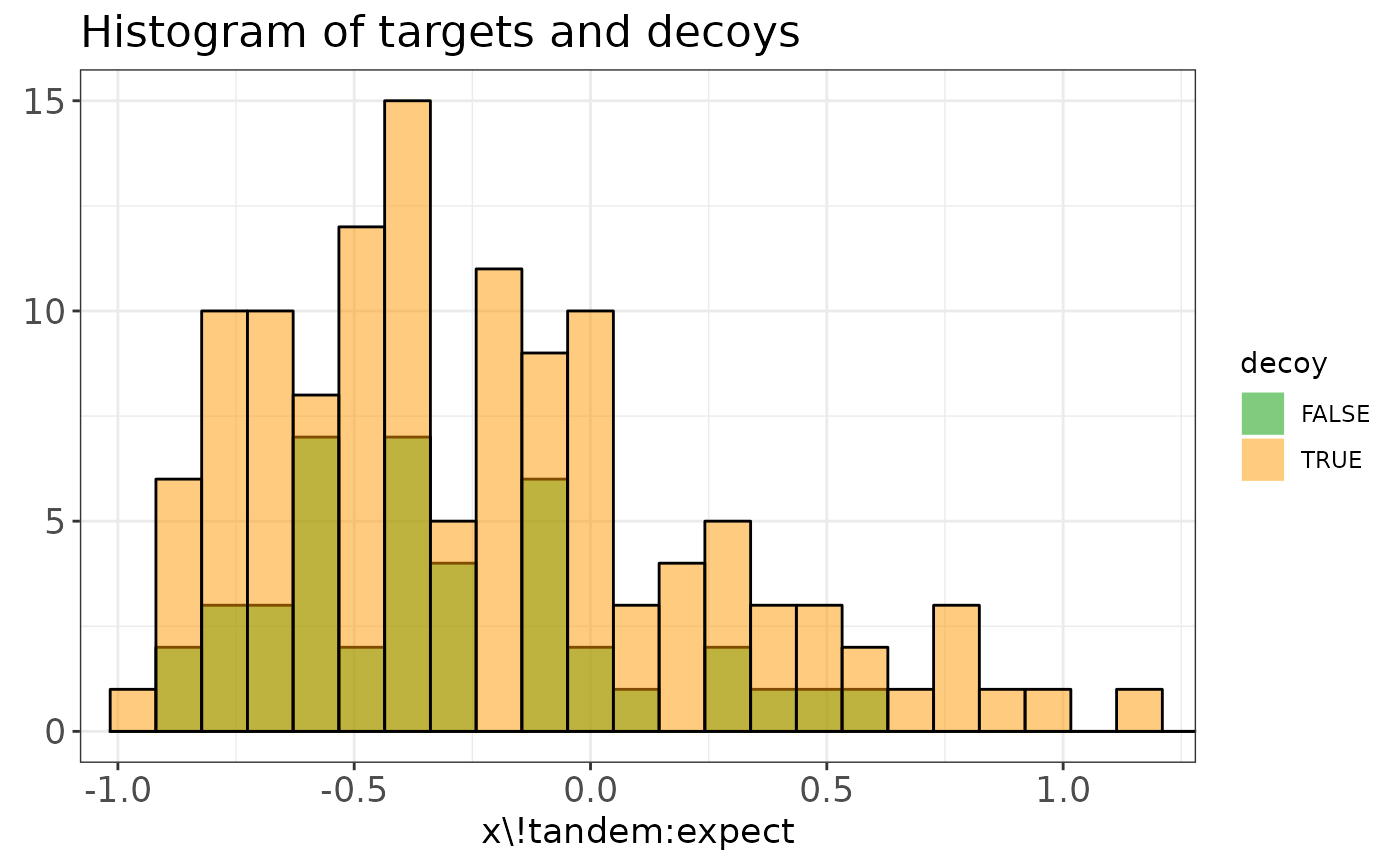## mzRident objects can also be used
library(mzR)